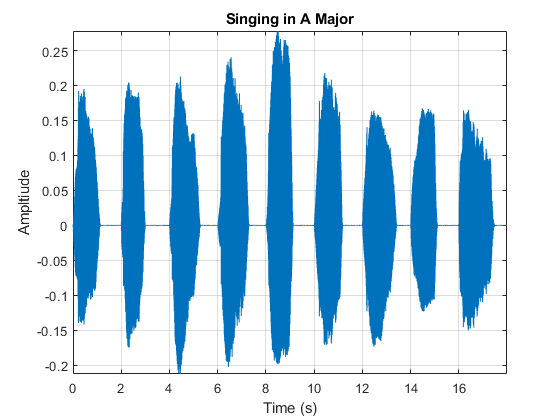pitchnn

Estimate pitch with deep learning neural network

Description

example

f0 = pitchnn(audioIn,fs) returns estimates of the fundamental frequency over time for audioIn with sample rate fs. Columns of the input are treated as individual channels.

f0 = pitchnn(audioIn,fs,Name,Value) specifies options using one or more Name,Value arguments. For example, f0 = pitchnn(audioIn,fs,'ConfidenceThreshold',0.5) sets the confidence threshold for each value of f0 to 0.5.

[f0,loc] = pitchnn(___) returns the time values, loc, associated with each fundamental frequency estimate.

[f0,loc,activations] = pitchnn(___) returns the activations of a crepe pretrained network.

pitchnn(___) with no output arguments plots the estimated fundamental frequency over time.

Examples

collapse all

Type crepe at the Command Window. If the Audio Toolbox model for CREPE is not installed, then the function provides a link to the location of the network weights. To download the model, click the link and unzip the file to a location on the MATLAB path.

crepeLocation = tempdir;
unzip(loc,crepeLocation)

Check that the installation is successful by typing crepe at the Command Window. If the network is installed, then the function returns a DAGNetwork (Deep Learning Toolbox) object.

crepe
ans =
DAGNetwork with properties:

Layers: [34×1 nnet.cnn.layer.Layer]
Connections: [33×2 table]
InputNames: {'input'}
OutputNames: {'pitch'}

The CREPE network requires you to preprocess your audio signals to generate buffered, overlapped, and normalized audio frames that can be used as input to the network. This example demonstrates the pitchnn function performing all of these steps for you.

Read in an audio signal for pitch estimation. Visualize and listen to the audio. There are nine vocal utterances in the audio clip.

soundsc(audioIn,fs)
T = 1/fs;
t = 0:T:(length(audioIn)*T) - T;
plot(t,audioIn);
grid on
axis tight
xlabel('Time (s)')
ylabel('Ampltiude')
title('Singing in A Major')Use the pitchnn function to produce the pitch estimate using a CREPE network with ModelCapacity set to tiny and ConfidenceThreshold disabled. Calling pitchnn with no output arguments plots the pitch estimation over time. If you call pitchnn before downloading the model, an error is printed to the Command Window with a download link.

pitchnn(audioIn,fs,'ModelCapacity','tiny','ConfidenceThreshold',0)With confidence thresholding disabled, pitchnn provides a pitch estimate for every frame. Increase the ConfidenceThreshold to 0.8.

pitchnn(audioIn,fs,'ModelCapacity','tiny','ConfidenceThreshold',0.8)Call pitchnn with ModelCapacity set to full. There are nine primary pitch estimation groupings, each group corresponding with one of the nine vocal utterances.

pitchnn(audioIn,fs,'ModelCapacity','full','ConfidenceThreshold',0.8)Call spectrogram and compare the frequency content of the signal with the pitch estimates from pitchnn. Use a frame size of 250 samples and an overlap of 225 samples or 90%. Use 4096 DFT points for the transform.

spectrogram(audioIn,250,225,4096,fs,'yaxis')Input Arguments

collapse all

Input signal, specified as a column vector or matrix. If you specify a matrix, pitchnn treats the columns of the matrix as individual audio channels.

Data Types: single | double

Sample rate of the input signal in Hz, specified as a positive scalar.

Data Types: single | double

Name-Value Arguments

Specify optional comma-separated pairs of Name,Value arguments. Name is the argument name and Value is the corresponding value. Name must appear inside quotes. You can specify several name and value pair arguments in any order as Name1,Value1,...,NameN,ValueN.

Example: pitchnn(audioIn,fs,'OverlapPercentage',50) sets the percent overlap between consecutive audio frames to 50.

Percentage overlap between consecutive audio frames, specified as a scalar in the range [0,100).

Data Types: single | double

Confidence threshold for each value of f0, specified as a scalar in the range [0,1).

To disable threshold, set this argument to 0.

Note

If the maximum value of the corresponding activations vector is less than 'ConfidenceThreshold', f0 is NaN.

Data Types: single | double

Model capacity, specified as 'tiny', 'small', 'medium', 'large', or 'full'.

Tip

'ModelCapacity' controls the complexity of the underlying deep learning neural network. The higher the model capacity, the greater the number of nodes and layers in the model.

Data Types: string | char

Output Arguments

collapse all

Estimated fundamental frequency in Hertz, returned as an N-by-C array, where N is the number of fundamental frequency estimates and C is the number of channels in audioIn.

Data Types: single

Time values associated with each f0 estimate, returned as a 1-by-N vector, where N is the number of fundamental frequency estimates. The time values correspond to the most recent samples used to compute the estimates.

Data Types: single | double

Activations from the CREPE network, returned as an N-by-360-by-C matrix, where N is the number of generated frames from the network and C is the number of channels in audioIn.

Data Types: single | double

 Kim, Jong Wook, Justin Salamon, Peter Li, and Juan Pablo Bello. “Crepe: A Convolutional Representation for Pitch Estimation.” In 2018 IEEE International Conference on Acoustics, Speech and Signal Processing (ICASSP), 161–65. Calgary, AB: IEEE, 2018. https://doi.org/10.1109/ICASSP.2018.8461329.

Audio Toolbox DocumentationTry MATLAB, Simulink, and Other Products

Get trial now